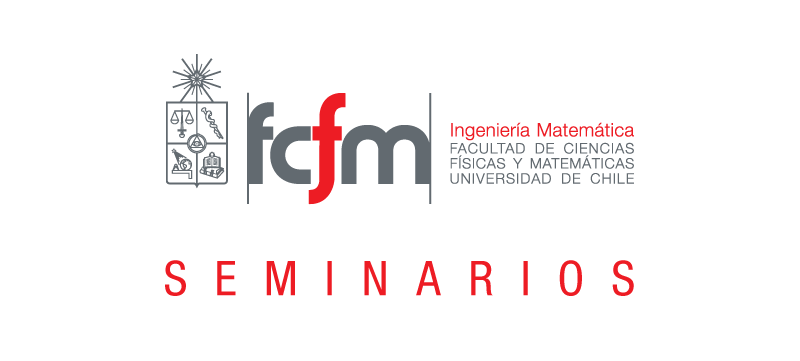Seleccionar páginaOptimization and Equilibrium Seminar

Speaker: Helmut Gfrefer

Affiliation: Institute of Computational Mathematics, Johannes Kepler University Linz, Austria

Title: Globalization of the SCD semismooth* Newton method in nonsmooth nonconvex optimization (joint work with J. Outrata)

Abstract:  We consider the problem of minimizing an lsc proper function. A locally superlinearly convergent method is given by the SCD (Subspace Containing Derivative) semismooth* Newton method for solving the first order necessary optimality conditions. We will discuss how to compute the SC derivative of the subdifferential mapping defining the linear system for the Newton direction. In order to globalize the SCD semismooth* Newton method, we combine it with some variant of the proximal gradient algorithm. We are able to show that every accumulation point of the sequence produced by our algorithm is S-stationary, and under some semismoothness* and regularity assumption, convergence is superlinear. Superlinear convergence is retained if the linear system defining the Newton direction is only approximately solved by the method of conjugate gradients. Some numerical examples are given.